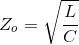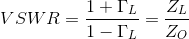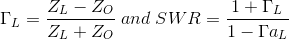# MCQs on Power Systems

##### Page 35 of 67. Go to page 1 2 3 4 5 6 7 8 9 10 11 12 13 14 15 16 17 18 19 20 21 22 23 24 25 26 27 28 29 30 31 32 33 34 35 36 37 38 39 40 41 42 43 44 45 46 47 48 49 50 51 52 53 54 55 56 57 58 59 60 61 62 63 64 65 66 67
01․ For distortion less line which of following relation are true
R/L = G/C.
R/L = C/G.
R = O, G = O.
None of above.

A distortionless line is one in which the attention constant α is independent of frequency while the phase constant is linearly dependent on frequency. For this line, the condition applicable is R/L = G/C or RC = LG.

02․ For a lossy transmission line, the characteristic impedance does not depend on
The operating frequency of the line.
The conductivity of conductors.
The permittivity of the dielectric separating the conductor.

A transmission line is said to be lossless if both its conductor and dielectric are lossless or R = O and G = O So characteristic impedance be,Inductance and capacitance don't depend on the load terminating the line.

03․ The characteristic impedance of a transmission line is
Directly proportional to its length.
Directly proportional to square root of its length.
Independent of its length.
Inversely proportional to square root of its length.

The characteristic impedance of a transmission line is given as,Where the inductance L and capacitance C are per unit lengths. These 'per unit length' values cancel out, and hence the characteristic impedance does not depend on the length of the transmission line.

04․ Which following statement are not true for line parameters R, L, G and C of transmission line
LC = μ ε and RG = σ ε.
G = 1/R where G is conductance of line.
Both R and G depend on the conductivity of conductor forming the line.
All of above.

For each line LC = μ ε and G/C = σ/ε,

05․ Which statement is not correct for the transmission line parameter R, L, G and C
At high frequencies at which most of communication system operate the internal inductance of the conductor is neglected.
The line parameter R,L,G and C are uniformly distributed along the entire length of the line C.
G = I/R. R is the AC resistance per unit length of conductor C.
For each line, the conductors are characterized by σ c, μ c, εo = ε c and the homog

Generally G = 1/R. But in AC transmission line G is not equal to 1/R, where G is conductance per unit length of the conductor. It is due to the presence of dielectric medium separating the conductor.

06․ The VSWR of transmission line is
Proportional to load and characteristic impedance.
Directly proportional to voltage minimum and inversely proportional to voltage maximum.
Directly proportional to load impedance and inversely to characteristic impedance.
Directly proportional to characteristic impedance and inversely to load impedance.07․ The SWR on a lossless transmission line of the characteristic impedance 100 ohm is 3. The line is the terminated by
A resistance of 100/3 ohm.
A resistance of 100 ohm.
A resistance of j300 ohm.
A resistance of j100/3 ohm.

For a transmission line, reflection co-efficient,Here ZL = 3 00 ΓL = 200/400 = ½ and SWR = 3.

08․ If R = 84 ohm/Km, G = 10-6 mho/Km, H = 0.01 H/Km, C = 0.061 μ F/Km and frequency = 1000 Hz, then what is the value of propagation constant of the transmission line
0.01 + j 0.02 per Km.
0.09 + j 0.018 per Km.
0.018 per Km.
None of them.

Z = R + jω L = 84 + j2π 100 × 0.01 = 84 + j 62.83 = 104.9 ∠ 36.8° ohm/Km. Y = G + jω C = 383.27 × 10-6 ∠ 89.85° mho/Km. So propagation constant by γ = √ (yz).

09․ For distortion less line which of following relation are true
R = O, G = O.
R/L = C/G.
R/L = G/C.
None of above.

A distortionless line is one in which the attention constant α is frequency independent while the phase constant is linearly dependent on frequency. For this line, the condition applicable is R/L = G/C.

10․ For a lossy transmission line, the characteristic impedance does not depend on
The operating frequency of the line.
The conductivity of conductors.
A transmission line is said to be lossless if both its conductor and dielectric are lossless or R = O and G = O So characteristic impedance be,Inductance and capacitance are not depends the load terminating the line.